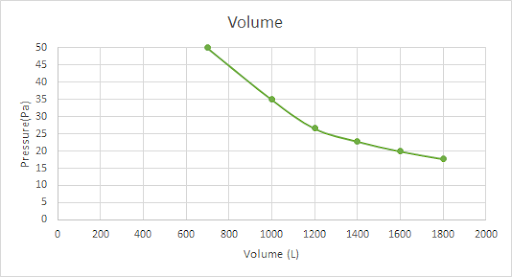# Question 52-Chemical and Physical Foundations of Biological Systems Practice Test for the MCAT

A diver with an oxygen tank is submerged $$30$$ m into the sea. At the moment his lungs are holding a volume of $$4$$ L, the estimated pressure at the initial depth is $$4$$ Bar. What volume will his lungs hold when the diver emerges to $$10$$ m and the pressure is around $$2$$ Bar? Consider constant temperature. (You may consult the attachment.)

The lungs are the main organs of the respiratory system of the human body. The lung’s function is to move fresh air into the human body, and remove the gases which are not beneficial to the body. During the breathing process, an exchange of gases is generated, inhaling air which contains oxygen, while taking out carbon dioxide.

The gas behaviors can be studied by the gas laws. These laws relate the pressure, volume, and temperature of a gas.

Boyle’s law relates the volume and the pressure of a gas, stating that at constant temperature the volume and the pressure vary inversely.

$P_1V_1=P_2V_2$

Charles’ law relates the volume and the temperature (absolute), stating that at constant pressure, the volume and the temperature vary proportionally.

$\dfrac{V_1}{T_1}=\dfrac{V_2}{T_2}$

Gay-Lussac’s law relates the pressure and the temperature, stating that at constant volume, the pressure and the temperature vary proportionally.

$\dfrac{P_1}{T_1} = \dfrac{P_2}{T_2}$

The temperature to work with these equations must be the absolute temperature. The unit used is Kelvin (K).

$T(K)=^\circ C+ 273.15$

The following graph shows the behavior of the pressure and the volume of a gas:Create a Profile